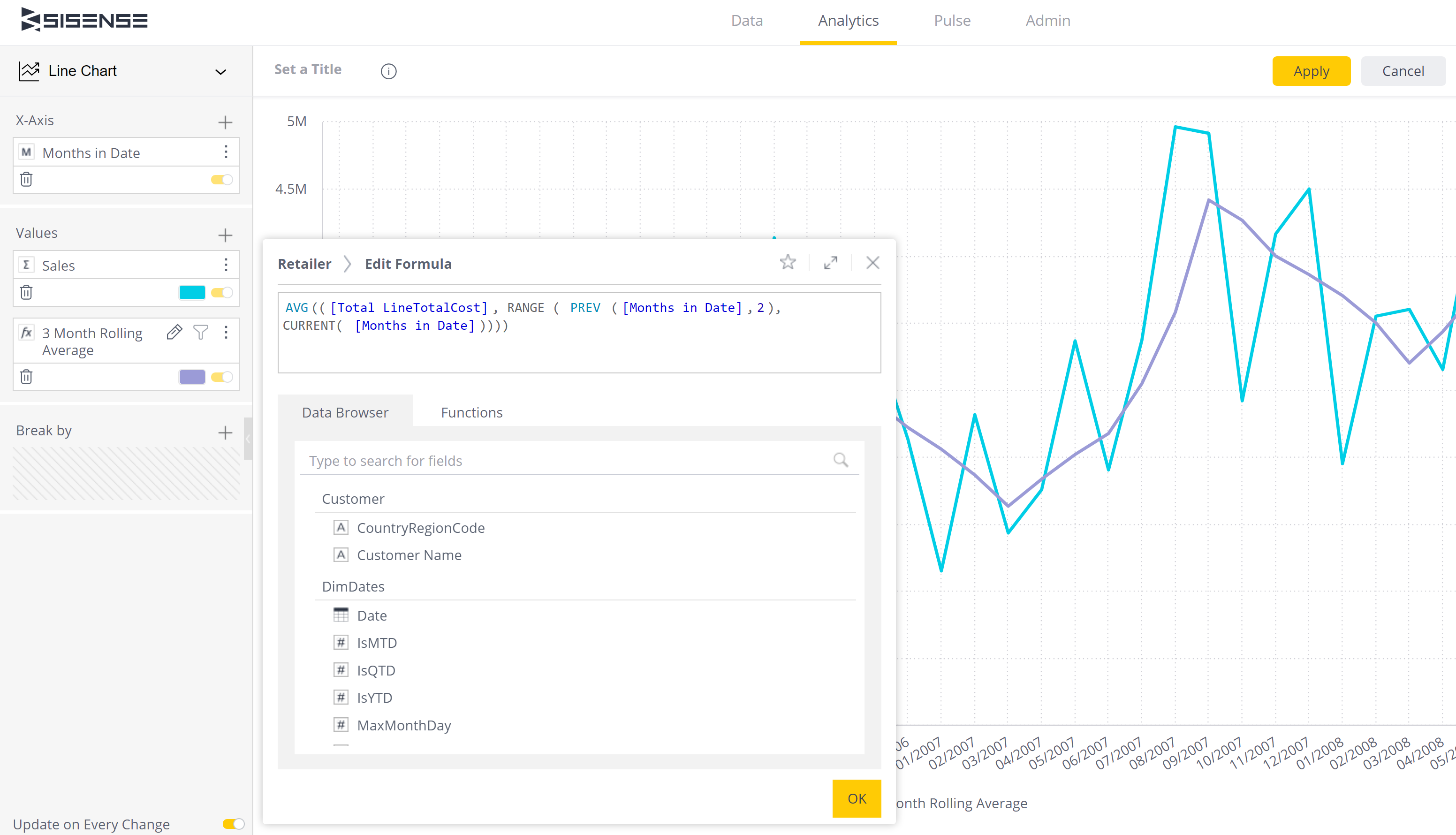Knowledgebase
Knowledgebase: Sisense Tips > DashboardsRolling average or relative date range on Dashboards / Widgets Posted by James Kruss on 19 October 2020 11:44 am Creating a rolling average or sum for a relative date range in a widget in Sisense is a common requirement.The solution is to use a formula using the Range function. The following assumes [Months in Date] is in the Rows. Where you see [Months in Date] in the formulas below, it is not a filter - just all items. The basic formula to sum a period of values looks like - SUM(([Total Sales], RANGE ( PREV ([Months in Date],12), PREV( [Months in Date],1 )))) This formula sums all the sales from 12 months prior to the relative date to 1 month prior to the relative date (12 months of sales offset by a month from now). The beauty of this is that it works on the date from the X axis or pivot rather than being relative to now. This could also be used for a 12 month rolling average by changing this to Average Sales (only change at the front of the formula; if you changed [Total Sales] to [Average Sales] you would be averaging each sale rather than sales for each month). AVG(([Total Sales], RANGE ( PREV ([Months in Date],12), PREV( [Months in Date],1 )))) If you wanted to include the current month, you could use the CURRENT function instead of Prev. It would look like - AVG(([Total Sales], RANGE ( PREV ([Months in Date],11), CURRENT( [Months in Date])))) Or a 3 month rolling average - AVG(([Total Sales], RANGE ( PREV ([Months in Date],2), CURRENT( [Months in Date])))) For any assistance with this contact RAPID BI.(2 vote(s)) Helpful Not helpful